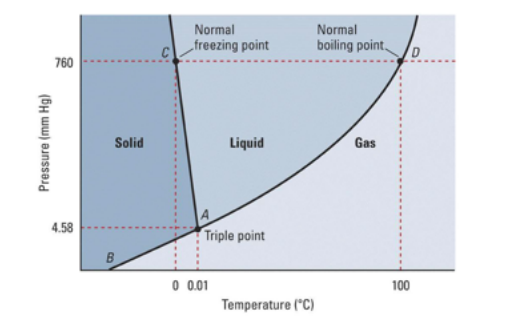# Problem: What does letter D represent?  a. fusion curve b. triple point c. sublimation point d. melting point e. critical point

🤓 Based on our data, we think this question is relevant for Professor Richter-Egger's class at UN OMAHA.

###### Problem Details

What does letter D represent?

a. fusion curve

b. triple point

c. sublimation point

d. melting point

e. critical pointWhat scientific concept do you need to know in order to solve this problem?

Our tutors have indicated that to solve this problem you will need to apply the Phase Diagram concept. You can view video lessons to learn Phase Diagram. Or if you need more Phase Diagram practice, you can also practice Phase Diagram practice problems.

What is the difficulty of this problem?

Our tutors rated the difficulty ofWhat does letter D represent?  a. fusion curve b. triple p...as medium difficulty.

How long does this problem take to solve?

Our expert Chemistry tutor, Sabrina took 1 minute and 29 seconds to solve this problem. You can follow their steps in the video explanation above.

What professor is this problem relevant for?

Based on our data, we think this problem is relevant for Professor Richter-Egger's class at UN OMAHA.